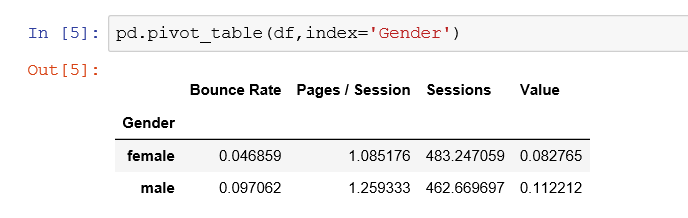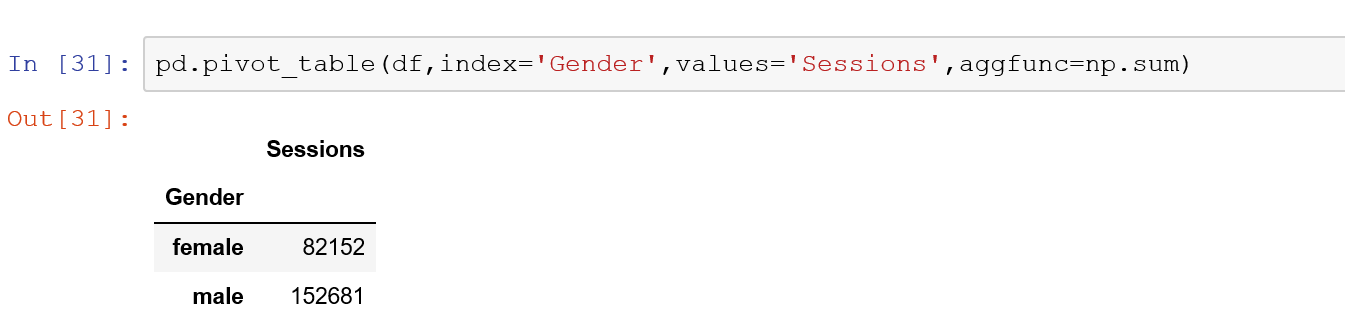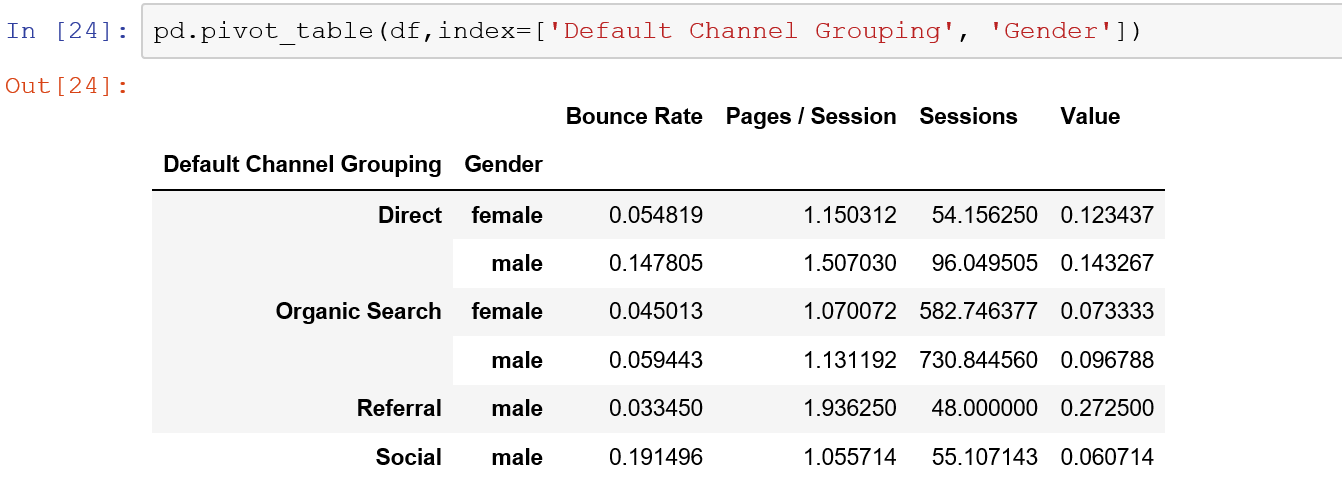# Create Pivot Tables with Pandas

One of the key actions for any data analyst is to be able to pivot data tables. Luckily Pandas has an excellent function that will allow you to pivot. To create this spreadsheet style pivot table, you will need two dependencies with is Numpy and Pandas. However, in newer iterations, you don’t need Numpy. Let’s open up Jupyter Notebooks using Google analytics as the dataset. You can find this dataset on the quickly improve your skills in Python by taking Python for Data Science Bootcamp Course.

For Video Instructions:

The essential parts of a pivot table are the following arguments:

• index
• values
• aggregation

We will explore to access these features using the Pandas library. The first step is to load the dataset. I am using my Google Analytics data for demo purposes.

### Import the Libraries:

To use the Pandas pivot table you will need Pandas and Numpy so let’s import these dependencies. We need Pandas to use the actual pivot table and Numpy will be used to handle the type of aggregation we want for the values in the table.

import pandas as pd
import numpy as np

We are using the standard aliases for both Pandas and Numpy which are pd and np.

Load the data set. My data is stored on a CSV file named categories which I exported from my Google Analytics.  I will load this data and store in a variable called df using the Pandas read_csv function.

`df = pd.read_csv('categories.csv')`

We can check the columns in the data by using the attribute columns at the end of our new data frame

`df.columns`

Index([‘Default Channel Grouping’, ‘Device Category’, ‘Gender’, ‘Affinity Category (reach)’, ‘Sessions’, ‘Pages / Session’, ‘Bounce Rate’, ‘Value’], dtype=’object’)

### Pandas Pivot Table

Now that we know the columns of our data we can start creating our first pivot table. We know that we want an index to pivot the data on. We can start with this and build a more intricate pivot table later.  We can use our alias pd with pivot_table function and add an index.

`pd.pivot_table(df,index='Gender')`This is known as a single index pivot. The next step would be a multi-index pivot table. However, the default aggregation for Pandas pivot table is the mean. We can change the aggregation and selected values by utilized other parameters in the function.

Using a single value in the pivot table.

`pd.pivot_table(df,index="Gender",values='Sessions", aggfunc = np.sum)`

Let’s take a look at the output.### Multi-Index Pandas Pivot Table

You can make multi-index pivot by just simply passing a list into the index parameter.

`pd.pivot_table(df,index=['Default Channel Grouping', 'Gender'])`Multi Aggregation Types in a Pandas Pivot Table

You can achieve this by simply adding a dictionary to your aggfunc parameter in the pivot table. Remember that dictionaries a signal by curly brackets and key value pairs. You can use the numpy function or simply write in the aggregation type with quotations.

```pd.pivot_table(df, index=['Gender', 'Default Channel Grouping'],values=['Sessions','Value'],aggfunc = {'Sessions':np.sum,'Value':np.median})

or

pd.pivot_table(df, index=['Gender', 'Default Channel Grouping'],values=['Sessions','Value'],aggfunc = {'Sessions':'sum','Value':'median'})

```

Percent of Grand Total in Pandas Pivot Table

To get a percent of grand total aggregation in your pivot table you will need to use a function that iterates across each row.  This is known as the lambda(). To  Luckily you can do this using a default lambda function in the aggfunc parameter.  For a full tutorial on Different Percent Types in Pandas Pivot Table.

`pd.pivot_table(df,index='Gender',values='Sessions',aggfunc = lambda x:x.sum()/df['Sessions'].sum())`For documentation check out the pydata pandas documents.#### Gaelim Holland

Subscribe
Notify of